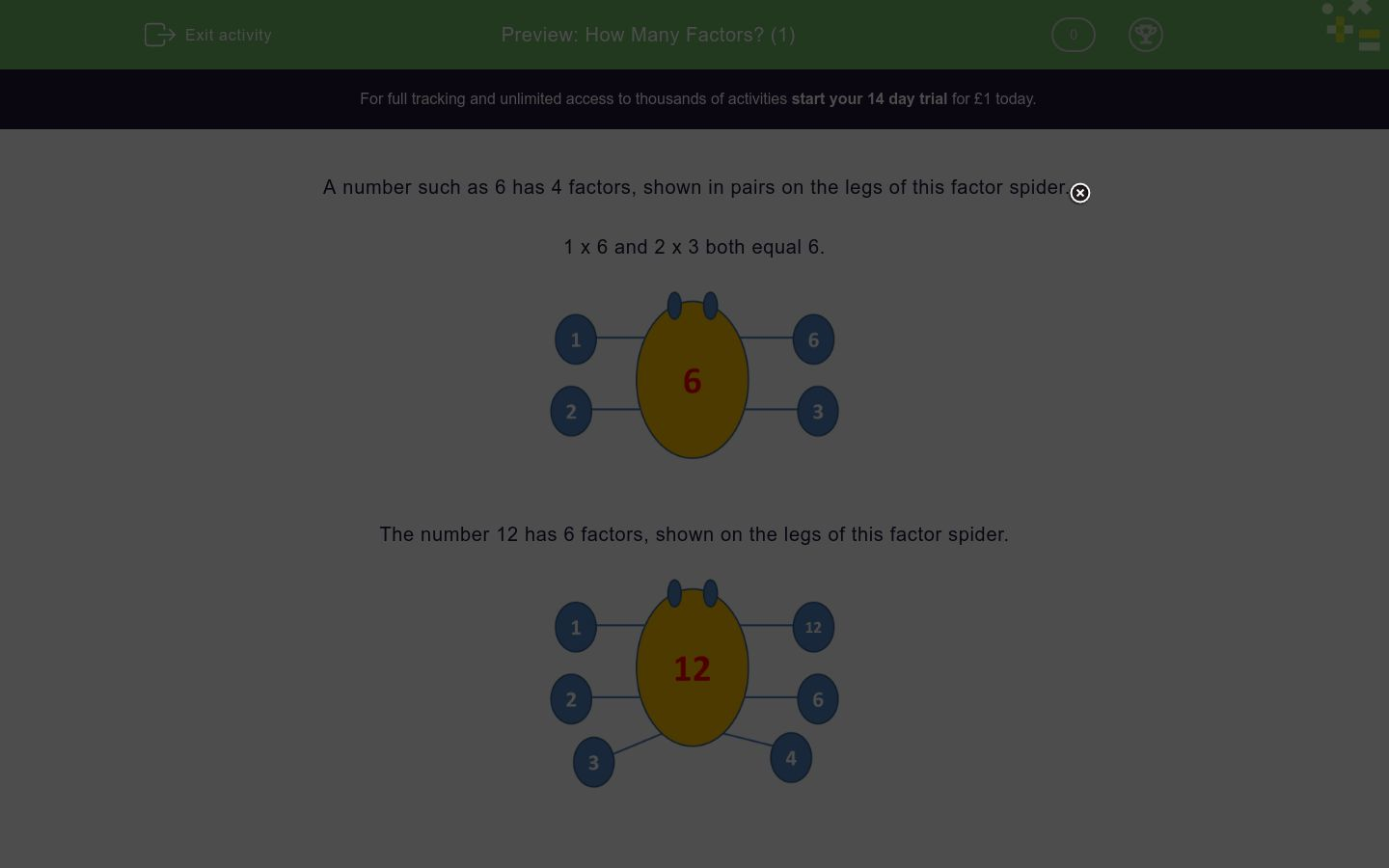# How Many Factors? (1)

In this worksheet, students state how many factors a number has by looking at its "factor spider"!Key stage:  KS 2

Curriculum topic:   Number: Multiplication and Division

Curriculum subtopic:   Identify Multiples and Factors

Difficulty level:### QUESTION 1 of 10

A number such as 6 has 4 factors, shown in pairs on the legs of this factor spider.

1 x 6 and 2 x 3 both equal 6.The number 12 has 6 factors, shown on the legs of this factor spider.Look at the factor spider for the number 9, which has 3 factors.

Note that the factor 3 should only be counted once.Want to understand this further and learn how this links to other topics in maths?

Why not watch this video?

How many factors does the following number have?

5

How many factors does the following number have?

6

How many factors does the following number have?

8

How many factors does the following number have?

3

How many factors does the following number have?

1

How many factors does the following number have?

4

How many factors does the following number have?

10

How many factors does the following number have?

14

How many factors does the following number have?

11

How many factors does the following number have?

12

• Question 1

How many factors does the following number have?

5

2
EDDIE SAYS
Prime 5: 1, 5
• Question 2

How many factors does the following number have?

6

4
EDDIE SAYS
6: 1, 2, 3, 6
• Question 3

How many factors does the following number have?

8

4
EDDIE SAYS
8: 1, 2, 4, 8
• Question 4

How many factors does the following number have?

3

2
EDDIE SAYS
Prime 3: 1, 3
• Question 5

How many factors does the following number have?

1

1
EDDIE SAYS
Square number
• Question 6

How many factors does the following number have?

4

3
EDDIE SAYS
Square number 4: 1, 2, 4
• Question 7

How many factors does the following number have?

10

4
EDDIE SAYS
10: 1, 2, 5, 10
• Question 8

How many factors does the following number have?

14

4
EDDIE SAYS
14: 1, 2, 7, 14
• Question 9

How many factors does the following number have?

11

2
EDDIE SAYS
Prime number 11: 1, 11
• Question 10

How many factors does the following number have?

12

6
EDDIE SAYS
12: 1, 2, 3, 4, 6, 12
---- OR ----